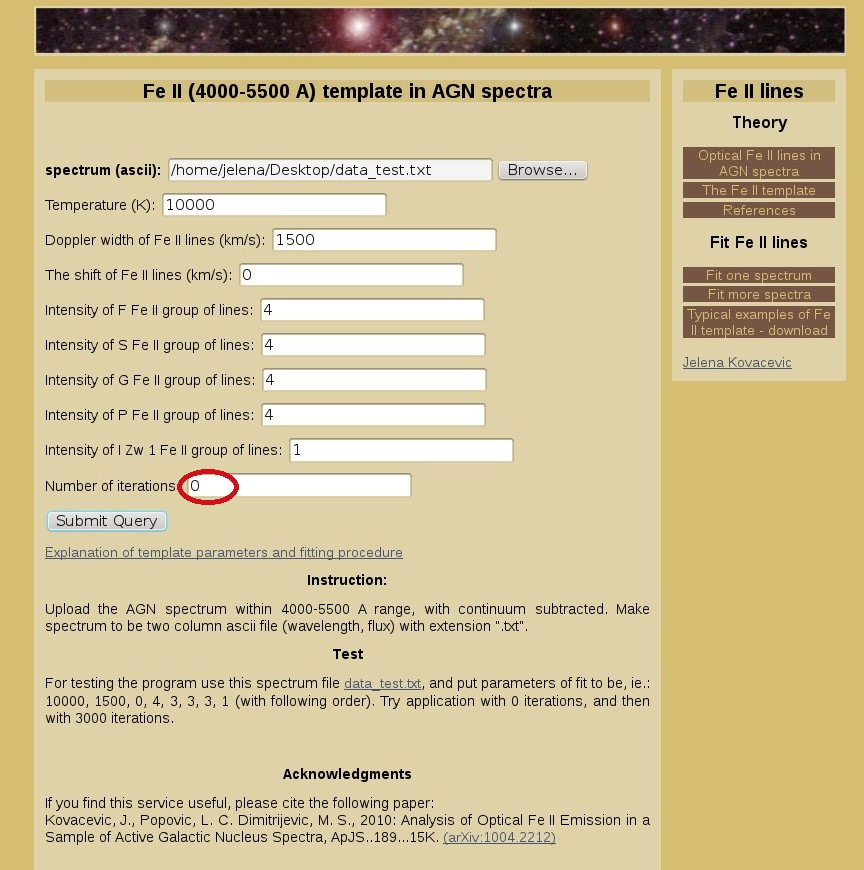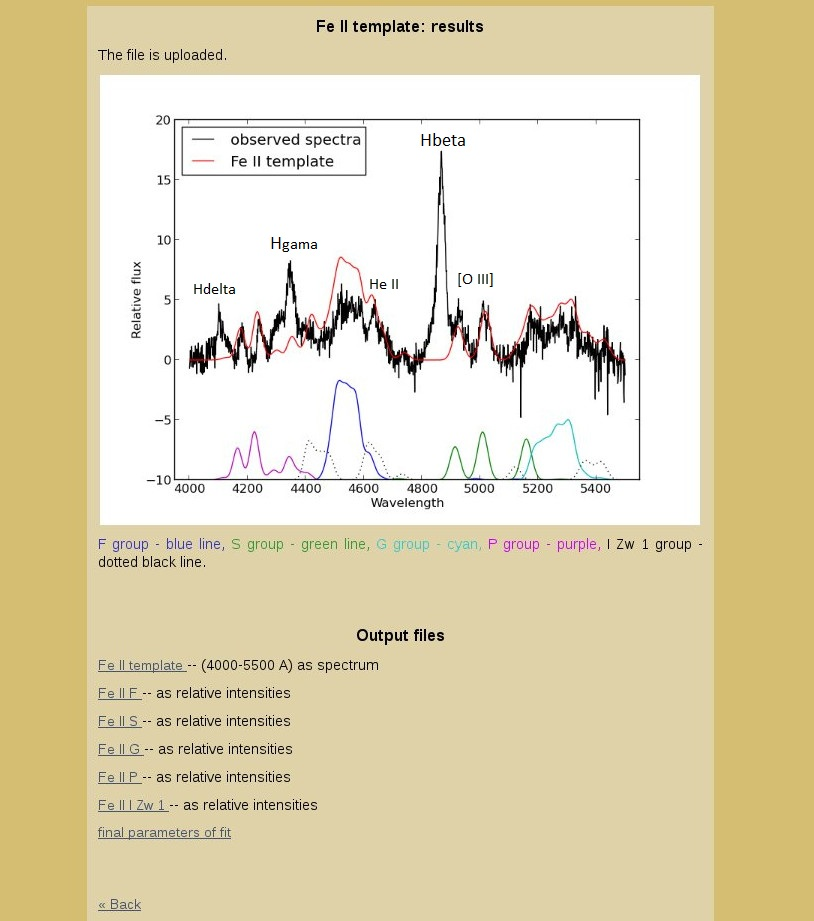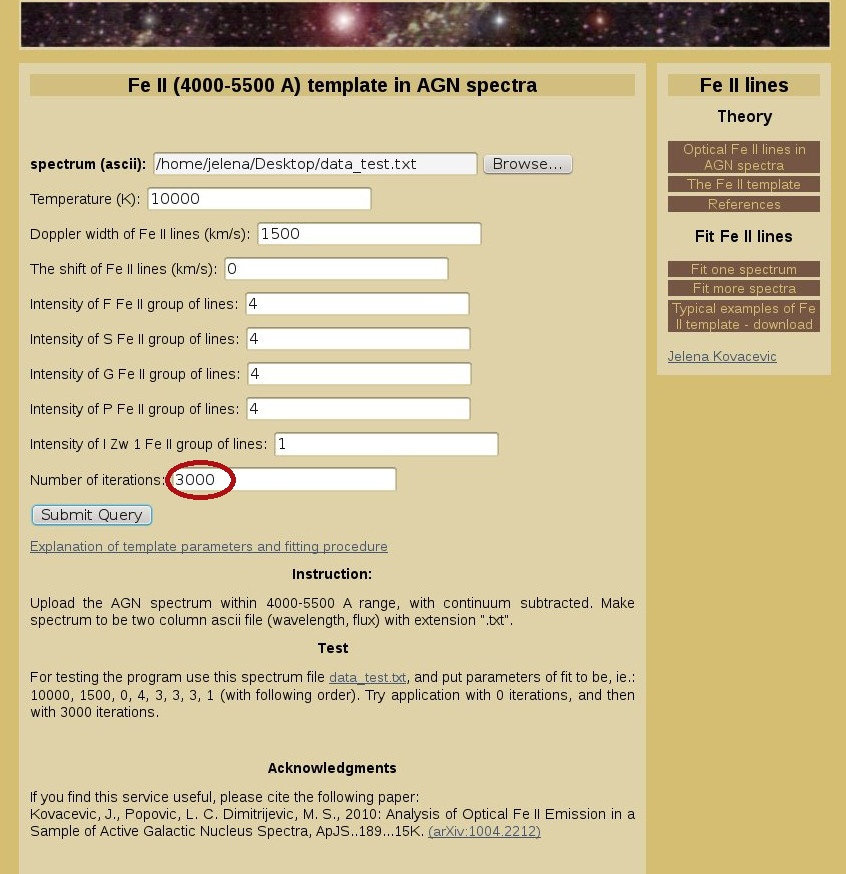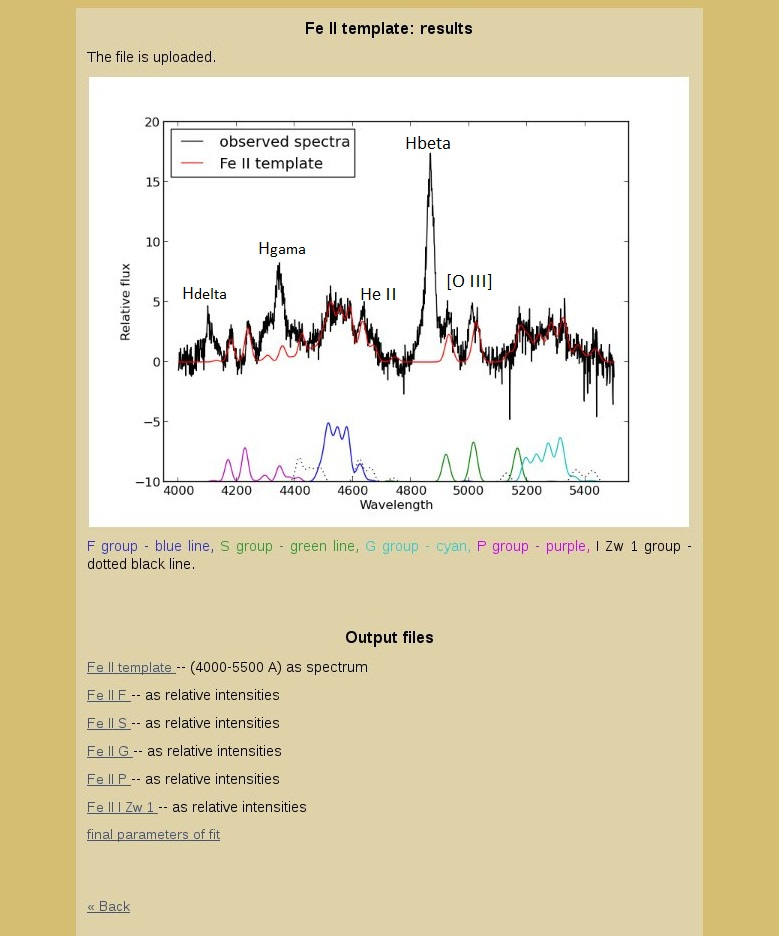# Fit one spectrum: explanation of template parameters and fitting procedure

To fit spectrum with Fe II template, first upload the ascii spectrum file (two columns, wavelength and flux), with extension ".txt". The spectrum should be continuum subtracted. The example of spectrum file is given in Test section: data_test.txt.

Than, fill the form with initial parameters.

Temperature:
The temperature is included in calculation of relative strengths of Fe II lines within one multiplet group (see here). Put 10000 K to be initial parameter.

Doppler width of Fe II lines (km/s):
We assume that all Fe II lines within considered range originate from the same AGN emission region. This implies the same physical and kinematical conditions in which these lines arise, i.e. the same width for all Fe II lines. For initial parameter you may put the approximate Doppler width of broad Hβ component (WDopp=FWHM/1.665).

The shift of Fe II lines (km/s):
Since we assume that all Fe II lines originate from the same emission region, their shift relative to narrow emission lines is the same. For initial parameter you may put 0.

Intensities of Fe II group of lines:
Each line group contains several Fe II lines, with common properties of transition (the same lower level of transition). The parameter of intensity represent the intensity of one of the lines within the group. The relative intensities of other lines from the group are calculated using the model. For P group that line is λ4233.172 Å, for F group it is λ4549.474 Å, for S λ5018.44 Å, for G λ5316.6 Å and for lines with relative intensities obtained from I Zw 1 it is: λ4660.593 Å.
Put initial intensities to be approximately the same order of magnitude as the flux in your spectrum.

Iterations:
Here is used χ2 minimization routine (Simplex method) for obtaining the best fit. With 0 iterations, no χ2 minimization routine will be applied. One should put at least 3000 iterations, to get the smallest value of χ2.

Each Fe II line is fitted with one Gaussian, described with parameter of width (W=WDoppo), parameter of shift (d=Δλ/λo), and intensity, calculated with formula (see here).
The Hβ, Hγ, Hδ, He II and [O III] lines, which overlap with Fe II lines in λλ4000-5400 Å range, are masked for fitting program, so program fits only the parts with pure Fe II lines (λλ4150-4250 Å, λλ4450-4600 Å, λλ5100-5500 Å). The Fe II lines which overlap with contaminating lines are connected with pure Fe II lines with calculated relative intensities.

## Output files

As output files it returns: total Fe II template (4000-5500 Å) in a form of spectrum. Each group of Fe II lines (F, S, G, P, I Zw 1) in the same form. Then, relative intensities of each Fe II line group separately, and the final parameters of fit.
File "Fe II template" may be used for Fe II subtraction in λλ4000-5400 Å range, or for the flux or EW calculation of Fe II lines. The same is for the files with Fe II line groups separately, in form of spectrum.
Files with Fe II line groups separately in form of relative intensities, may be used for automatic fitting of similar spectra. Namely, the line intensities should be convoluted with Gaussians (with the same shift and width). Than, the spectra should be fitted with 7 free parameters: intensities for each line group (5), width and shift. It is recommended to mask the contaminating lines in observed range, to simplified the procedure.

## One example

Figure 1 Submit the file and initial parameters. If number of iterations is 0, the obtained picture and output files will be calculated by given initial parameters (see Figure 2).Figure 2Figure 3 If number of iterations is 3000 (or more), the obtained picture and output files will be calculated with the parameters obtained from the best fit, using χ2 minimization routine (see Figure 4).Figure 4Final parameters of fit:
Temperature (K): 10228.496094
Doppler width of Fe II lines (km/s): 1075.674586
The shift of Fe II lines (km/s): 397.036323
Intensity of F Fe II group of lines: 2.917780
Intensity of S Fe II group of lines: 3.314444
Intensity of G Fe II group of lines: 3.220845
Intensity of P Fe II group of lines: 2.852378
Intensity of I Zw 1 Fe II group of lines: 0.669535
chisq= 443.537540

« Home